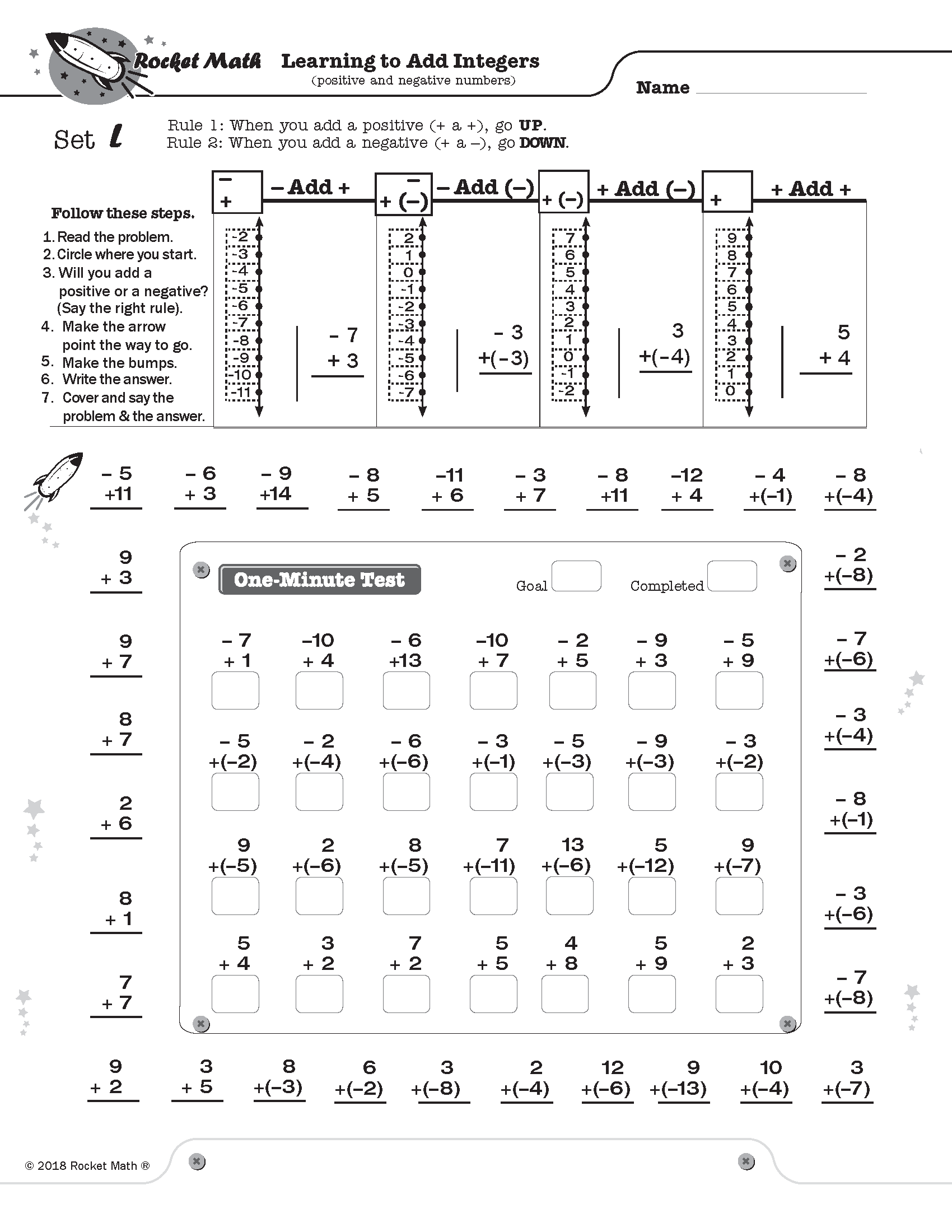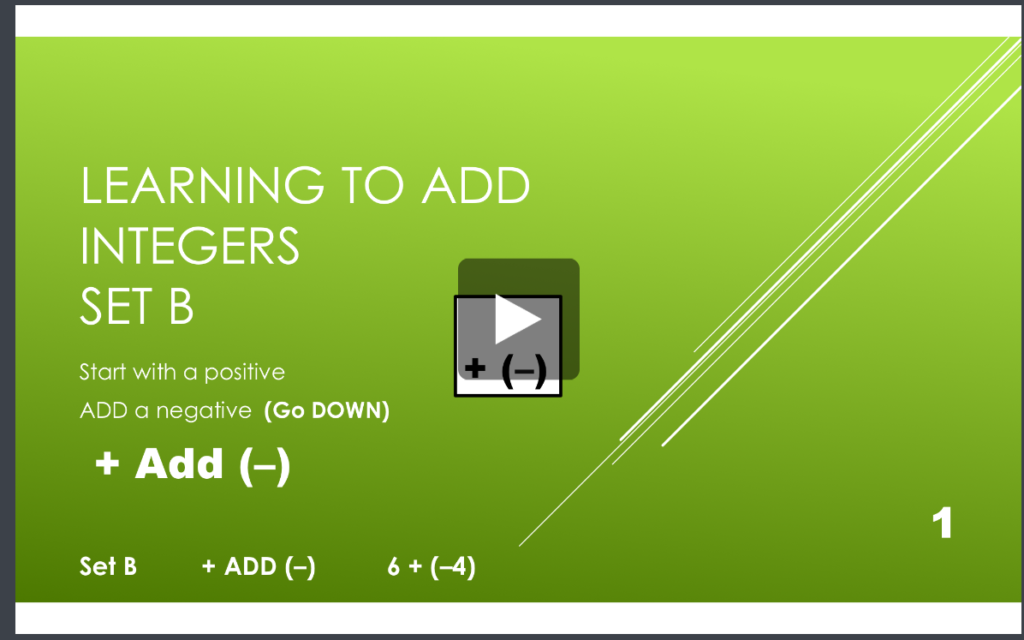### Four Problem Types to LearnLearning to Add Integers displays problems on a vertical number line and then teaches students two rules about how to solve problems that add positive and negative numbers.
Rule 1:  When you add a positive number, go UP.
Rule 2:  When you add a negative number, go DOWN.

Click to see online lesson.  Doing problems on the vertical number line is more intuitively appealing because UP is more and DOWN is always less.  This makes crossing zero a little easier to comprehend.

Students learn how these two rules play out with two types of problems: when starting with a positive number and when starting with a negative number. Students gradually learn all four types of problems.  On each worksheet they see how to solve each problem type using the number line working with their partner.  Then students learn to recognize the pattern of each problem type by orally answering several examples of each type with their partner (going around the outside of the page).  You will probably not be surprised that there is a one-minute test on each set.   Students are to be 100% accurate and to meet or beat their goal from the special writing speed test for Learning to Add integers (the fastest goal is only 28 problems in a minute).

Students can watch 4 online lessons which teach how each type of problem is solved and why it is correct.